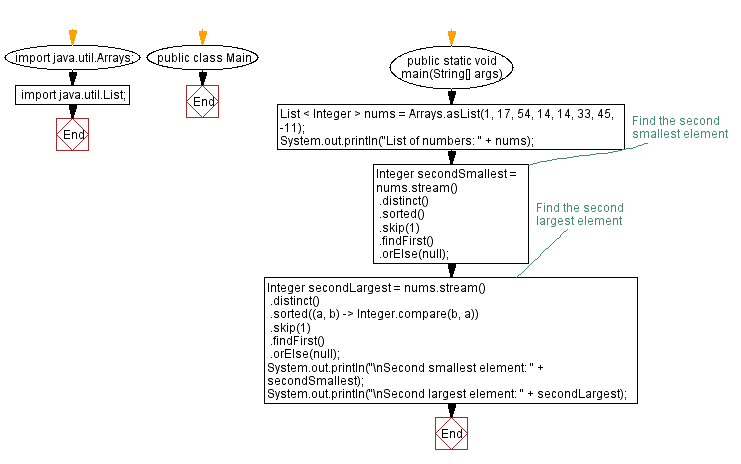﻿ Java Program: Find Second Smallest and Largest Elements in List of Integers using Streams

# Java Program: Find Second Smallest and Largest Elements in a List of Integers using Streams

## Java Stream: Exercise-8 with Solution

Write a Java program to find the second smallest and largest elements in a list of integers using streams.

Sample Solution:

Java Code:

``````import java.util.Arrays;
import java.util.List;

public class Main {
public static void main(String[] args) {
List < Integer > nums = Arrays.asList(1, 17, 54, 14, 14, 33, 45, -11);
System.out.println("List of numbers: " + nums);
// Find the second smallest element
Integer secondSmallest = nums.stream()
.distinct()
.sorted()
.skip(1)
.findFirst()
.orElse(null);

// Find the second largest element
Integer secondLargest = nums.stream()
.distinct()
.sorted((a, b) -> Integer.compare(b, a))
.skip(1)
.findFirst()
.orElse(null);

System.out.println("\nSecond smallest element: " + secondSmallest);
System.out.println("\nSecond largest element: " + secondLargest);
}
}
```
```

Sample Output:

```List of numbers: [1, 17, 54, 14, 14, 33, 45, -11]

Second smallest element: 1

Second largest element: 45
```

Explanation:

In the above exercise,

We create a list of integers (numbers) and use streams to find the second smallest element by applying distinct, sorting the elements in ascending order, skipping the first element, and locating the first element. Similarly, we find the second largest element by sorting the elements in descending order. The orElse method handles the case when the list is empty or there are no second smallest/largest elements.

Flowchart:Java Code Editor:

Improve this sample solution and post your code through Disqus

What is the difficulty level of this exercise?

Test your Programming skills with w3resource's quiz.

﻿

## Java: Tips of the Day

Converts a string from camelcase:

```public static String fromCamelCase(String input, String separator) {
return input
.replaceAll("([a-z\\d])([A-Z])", "\$1" + separator + "\$2")
.toLowerCase();
}
```

Ref: https://bit.ly/3u09A9E

We are closing our Disqus commenting system for some maintenanace issues. You may write to us at reach[at]yahoo[dot]com or visit us at Facebook